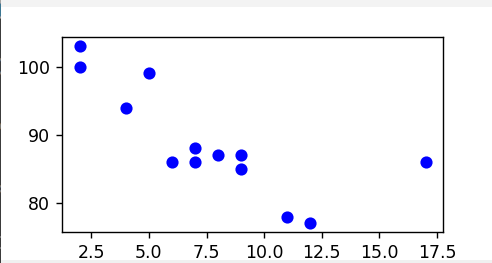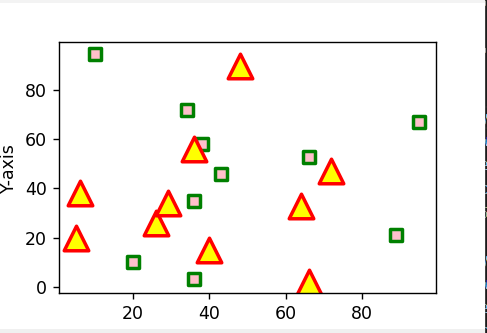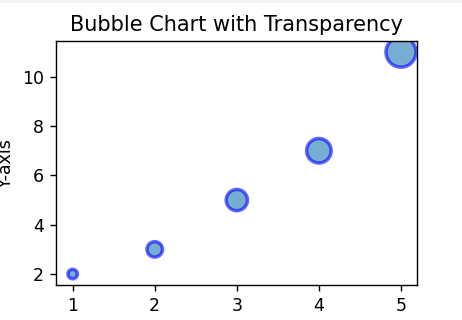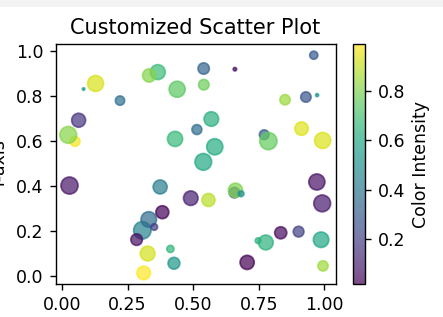# matplotlib.pyplot.scatter() in Python

Matplotlib stands as an extensive library in Python, offering the capability to generate static, animated, and interactive visualizations. The Matplotlib.pyplot.scatter() in Python extends to creating diverse plots such as scatter plots, bar charts, pie charts, line plots, histograms, 3-D plots, and more.

For a more in-depth understanding, additional information can be found in the guide titled “Python Matplotlib – An Overview.”

## What is Matplotlib.pyplot.scatter()?

The matplotlib.pyplot.scatter() plots serve as a visual tool to explore and analyze the relationships between variables, utilizing dots to depict the connection between them. The matplotlib library provides the scatter() method, specifically designed for creating scatter plots. These plots are instrumental in illustrating the interdependencies among variables and how alterations in one variable can impact another

Syntax: matplotlib.pyplot.scatter(x_axis_data, y_axis_data, s=None, c=None, marker=None, cmap=None, vmin=None, vmax=None, alpha=None, linewidths=None, edgecolors=None)

Parameters:

• `x_axis_data`: An array containing data for the x-axis.matplotlib
• `s`: Marker size, which can be a scalar or an array of size equal to the size of x or y.
• `c`: Color of the sequence of colors for markers.
• `marker`: Marker style.
• `cmap`: Colormap name.
• `linewidths`: Width of the marker border.
• `edgecolor`: Marker border color.
• `alpha`: Blending value, ranging between 0 (transparent) and 1 (opaque).

Except for `x_axis_data` and `y_axis_data`, all other parameters are optional, with their default values set to None. The scatter plot examples below demonstrate the versatility of the scatter() method by showcasing various combinations of these optional parameters.

## Matplotlib.pyplot.scatter() in Python

There are various ways of creating plots using matplotlib.pyplot.scatter() in Python, There are some examples that illustrate the matplotlib. pyplot.scatter() function in matplotlib.plot:

• Basic Scatter Plot
• Scatter Plot With Multiple Datasets
• Bubble Chart Plot
• Customized Scatter Plot

### Scatter Plot in Matplotlib

By importing matpltlib. plot () we created a scatter plot. It defines x and y coordinates, then plots the points in blue and displays the plot.

## Python3

 `import` `matplotlib.pyplot as plt`   `x ``=``[``5``, ``7``, ``8``, ``7``, ``2``, ``17``, ``2``, ``9``,` `    ``4``, ``11``, ``12``, ``9``, ``6``] `   `y ``=``[``99``, ``86``, ``87``, ``88``, ``100``, ``86``, ` `    ``103``, ``87``, ``94``, ``78``, ``77``, ``85``, ``86``]`   `plt.scatter(x, y, c ``=``"blue"``)`   `# To show the plot` `plt.show()`

Output :Basic Scatter Plot

### Plot Multiple Datasets on a Scatterplot

The below code generates a scatter plot showcasing two distinct datasets, each with its set of x and y coordinates. The code employs different markers, colors, and styling options for enhanced visualization.

## Python3

 `import` `matplotlib.pyplot as plt`   `# dataset-1` `x1 ``=` `[``89``, ``43``, ``36``, ``36``, ``95``, ``10``, ` `      ``66``, ``34``, ``38``, ``20``]`   `y1 ``=` `[``21``, ``46``, ``3``, ``35``, ``67``, ``95``, ` `      ``53``, ``72``, ``58``, ``10``]`   `# dataset2` `x2 ``=` `[``26``, ``29``, ``48``, ``64``, ``6``, ``5``,` `      ``36``, ``66``, ``72``, ``40``]`   `y2 ``=` `[``26``, ``34``, ``90``, ``33``, ``38``, ` `      ``20``, ``56``, ``2``, ``47``, ``15``]`   `plt.scatter(x1, y1, c ``=``"pink"``, ` `            ``linewidths ``=` `2``, ` `            ``marker ``=``"s"``, ` `            ``edgecolor ``=``"green"``, ` `            ``s ``=` `50``)`   `plt.scatter(x2, y2, c ``=``"yellow"``,` `            ``linewidths ``=` `2``,` `            ``marker ``=``"^"``, ` `            ``edgecolor ``=``"red"``, ` `            ``s ``=` `200``)`   `plt.xlabel(``"X-axis"``)` `plt.ylabel(``"Y-axis"``)` `plt.show()`

Output :Scatter Plot with Multiple Datasets

### Bubble Plots in Matplotlib

This code generates a bubble chart using Matplotlib. It plots points with specified x and y coordinates, each represented by a bubble with a size determined by the `bubble_sizes` list. The chart has customization for transparency, edge color, and linewidth. Finally, it displays the plot with a title and axis labels.

## Python3

 `import` `matplotlib.pyplot as plt`   `# Data` `x_values ``=` `[``1``, ``2``, ``3``, ``4``, ``5``]` `y_values ``=` `[``2``, ``3``, ``5``, ``7``, ``11``]` `bubble_sizes ``=` `[``30``, ``80``, ``150``, ``200``, ``300``]`   `# Create a bubble chart with customization` `plt.scatter(x_values, y_values, s``=``bubble_sizes, alpha``=``0.6``, edgecolors``=``'b'``, linewidths``=``2``)`   `# Add title and axis labels` `plt.title(``"Bubble Chart with Transparency"``)` `plt.xlabel(``"X-axis"``)` `plt.ylabel(``"Y-axis"``)`   `# Display the plot` `plt.show()`

Output :Bubble Chart

### Custom a Matplotlib Scatterplot

By importing Matplotlib we create a customized scatter plot using Matplotlib and NumPy. It generates random data for x and y coordinates, colors, and sizes. The scatter plot is then created with customized properties such as color, size, transparency, and colormap. The plot includes a title, axis labels, and a color intensity scale. Finally, the plot is displayed

## Python3

 `import` `matplotlib.pyplot as plt` `import` `numpy as np`   `# Generate random data` `x ``=` `np.random.rand(``50``)` `y ``=` `np.random.rand(``50``)` `colors ``=` `np.random.rand(``50``)` `sizes ``=` `100` `*` `np.random.rand(``50``)`   `# Create a customized scatter plot` `plt.scatter(x, y, c``=``colors, s``=``sizes, alpha``=``0.7``, cmap``=``'viridis'``)`   `# Add title and axis labels` `plt.title(``"Customized Scatter Plot"``)` `plt.xlabel(``"X-axis"``)` `plt.ylabel(``"Y-axis"``)`   `# Display color intensity scale` `plt.colorbar(label``=``'Color Intensity'``)`   `# Show the plot` `plt.show()`

Output :Customized Scatter Plot

### Conclusion

In conclusion, `matplotlib.pyplot.scatter()` Python is a versatile and powerful tool for visualizing relationships between variables through scatter plots. Its flexibility allows for the customization of markers, colors, sizes, and other properties, providing a dynamic means of representing complex data patterns. Whether for basic exploratory analysis or detailed data interpretation, this function plays a crucial role in creating informative and visually appealing scatter plots within the Python programming environment.

Whether you're preparing for your first job interview or aiming to upskill in this ever-evolving tech landscape, GeeksforGeeks Courses are your key to success. We provide top-quality content at affordable prices, all geared towards accelerating your growth in a time-bound manner. Join the millions we've already empowered, and we're here to do the same for you. Don't miss out - check it out now!

Previous
Next